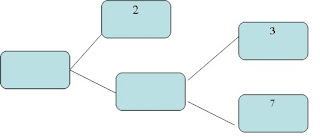## Monday, March 29, 2010

### Assignment-Real numbers

Q1:Show that every positive even integer is of the form 2q and that every positive odd integer is of the form 2q+1 for some integer q.
Q2:Show that every positive odd integer is of the form 4q+1or 4q+3 for some integer q.
Q3:Show that every positive even integer is of the form 4q or 4q+2 for some integer q.
Q4.Show that every positive odd integer is of the form 6q+1 or 6q+3 or 6q+5 for some integer q.
Q5:Show that one and only one out of n,n+2,n+4 is divisible by 3 ,where n is any positive integer.
Q6: Prove that one of every three consecutive positive integers is divisible by 3.
Q7:If H.C.F(72,120)=24 then what is LCM(72,120)?
Q8:If LCM of two numbers is 2079 and their HCF is 27. If one of the numbers is 297.Find the other number.
Q9:Find HCF of 42,72,108 by Euclids Division Lemma.
Q10.Under what conditions the decimal expansion of a rational number terminates?
Q11:Find the largest number which divides 2053 and 967 leaving remainder 5 and 7 respectively?
Q12:Find the greatest number which exactly divides 285 and 1249 leaving remainders 9 and 7 respectively.
Q13:Two tankers contain 850 litres and 680 litres of petrol respectively.Find the maximum capacity of a container which can measure the petrol of either tanker in exact number of times.
Q14.Prove that √3 +√7 is irrational.
Q15:Prove that 5-√3 is irrational.
Q16:Without actual division find whether the rational number 15/1600 is a terminating or non-terminating repeating decimal.
Q17: Prove that 17 × 41 × 43 × 61 + 43 is a composite number.
Q18.Find the H.C.F of 81 and 237 and express it as a linear combination of 81 and 237.
Q19.If the H.C.f of 210 and 55 is expressible in the form 210 x 5+55 y,find y.
Q20.Complete the missing entries in the following factor tree.